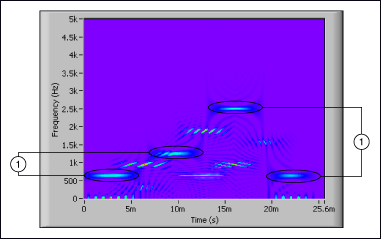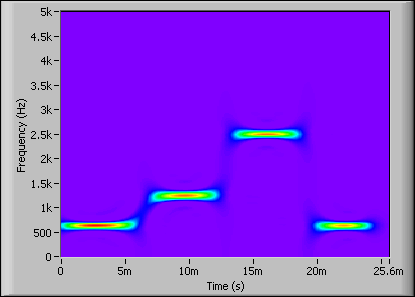# Gabor Spectrogram (Advanced Signal Processing Toolkit)

LabVIEW 2014 Advanced Signal Processing Toolkit Help

Edition Date: June 2014

Part Number: 372656C-01

»View Product InfoDownload Help (Windows Only)

Because you can decompose a signal as a linear combination of a family of elementary functions, you can consider the Wigner-Ville Distribution (WVD) of a signal to be the summation of the WVD of each elementary function, or auto-term, and the cross-terms of each pair of elementary functions.

You can use the Gabor spectrogram method to reduce the cross-term interference of the WVD. This method decomposes a signal with the Gabor expansion and then sums the WVD of each auto-term with some cross-terms. Because the Gabor spectrogram method uses the Gabor expansion first and then the WVD, the Gabor spectrogram also is called the Gabor expansion-based spectrogram. The Gabor spectrogram also is called the time-frequency distribution series. The Gabor spectrogram is a method unique to the LabVIEW Time Frequency Analysis Tools.

The Gabor spectrogram has a better time-frequency resolution than the short-time Fourier transform (STFT) spectrogram method and less cross-term interference than the WVD method. The Gabor spectrogram algorithm includes simple analytic forms, and you can implement the Gabor spectrogram with interpolation filters. Therefore, the Gabor spectrogram is more efficient than the Choi-Williams Distribution (CWD) and the Cone-Shaped Distribution (CSD), especially with large signal lengths. However, the Gabor spectrogram usually requires more computation time than the STFT spectrogram or the WVD.

Use TFA Fast Gabor Spectrogram VI to compute the Gabor spectrogram.

## Selecting an Appropriate Gabor Spectrogram Order and Window Length

You need to select the order of the Gabor spectrogram and the window length of the Gabor spectrogram properly to balance the time-frequency resolution and the cross-term interference. For Gabor spectrograms with a low order, the window length affects the time resolution, the frequency resolution, and the cross-term interference of the Gabor spectrogram. As in the case of the STFT, a narrow window length results in a coarse frequency resolution, a fine time resolution, and severe cross-term interference between two auto-terms with the same time center. A wide window length results in a fine frequency resolution, a coarse time resolution, and severe cross-term interference between two auto-terms with the same frequency center. The selection of the window length in the Gabor spectrogram is much less sensitive than in the STFT spectrogram. Also, regardless of the window length you select, the Gabor spectrogram always converges to the WVD as the order increases.

The order of the Gabor spectrogram balances the time-frequency resolution and the cross-term interference of the Gabor spectrogram. As the order increases, the time-frequency resolution of the Gabor spectrogram improves, but the spectrogram includes more cross-term interference and requires a longer computation time. When the order is zero, the Gabor spectrogram is non-negative and is similar to the STFT spectrogram. As the order increases, the Gabor spectrogram converges to the WVD. For most real-world applications, choose an order of two to five to balance the time-frequency resolution and cross-term suppression.

The following figure shows the Gabor spectrogram of the example frequency hopper signal when the order is 0 and the window length is 128.Notice that the signal in the previous figure is similar to the STFT spectrogram in the example frequency hopper signal with window length of 128. The Gabor spectrogram of the example frequency hopper signal in the previous figure has a coarse time-frequency resolution and does not include cross-term interference.

The following figure shows the Gabor spectrogram of the example frequency hopper signal when the order is 20 and the window length is 128.1 Auto-terms

The signal in the previous figure is similar to the WVD of the example frequency hopper signal because the Gabor spectrogram converges to the WVD as the order increases. The Gabor spectrogram of the example frequency hopper signal in the previous figure has a fine time-frequency resolution and includes cross-term interference.

The following figure shows the Gabor spectrogram of the example frequency hopper signal when the order is 3 and the window length is 128.The signal in the previous figure has a higher time-frequency resolution than the STFT spectrogram and less cross-term interference than the WVD.

The following figure shows a signal with three Gaussian components. The composite Gaussian signal can be a useful test signal for testing the time-frequency resolution and the cross-term interference of a time-frequency analysis algorithm because the composite Gaussian signal has compact signal components that should be very narrow on the time-frequency plane.The example signal in the previous figure contains one Gaussian-windowed linear chirp signal and two Gaussian-windowed sine wave signals. The linear chirp has the same time center as one of the sine waves and the same frequency center as the other sine wave, but you cannot see that in the time-domain representation in the previous figure.

The following figure shows the Gabor spectrogram of the composite Gaussian signal example when the order is 5 and the window length is 32.1 Auto-terms

The following figure shows the Gabor spectrogram of the composite Gaussian signal example when the order is 5 and the window length is 256.1 Auto-terms

Notice in the previous two figures that the cross-term interference along the time axis increases with the window length and the cross-term interference along the frequency axis decreases with the window length.

Not Helpful